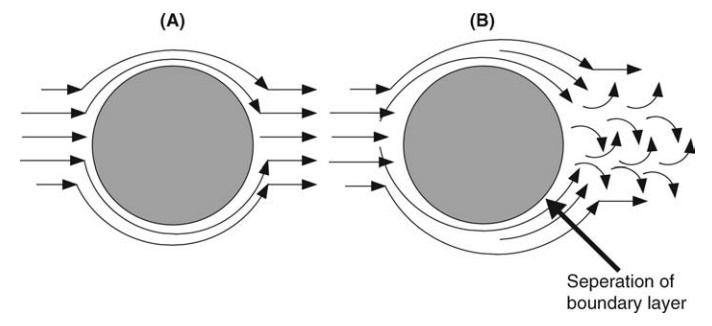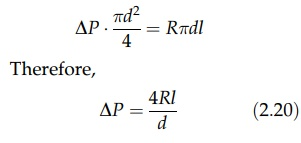# Calculation of the Pressure Drop in a Pipe Due to Friction

| Home | | Pharmaceutical Technology |

## Chapter: Pharmaceutical Engineering: Fluid Flow

Flow of fluid past a cylinder (A) laminar and (B) turbulent.

CALCULATION OF THE PRESSURE DROP IN A PIPE DUE TO FRICTION

If the volumetric flow rate of a liquid of density ρ and viscosity η through a pipe of diameter d is Q, the derivation of the mean velocity, u, from the flow rate and pipe area completes the data required for calculating Re.FIGURE 2.11 Flow of fluid past a cylinder (A) laminar and (B) turbulent.

If the pipe roughness factor is known, the equivalent value of R/ρu2 can be determined from Figure 2.10, and the shear stress at the pipe wall can be calculated. The total frictional force opposing motion is the product of R and the surface area of the pipe, πdl, where l is the length of the pipe. If the unknown pressure drop across the pipe is DP, the force driving through the pipe is ΔP · πd2/4. Equating pressure force and frictional force givesDivision by ρg gives the pressure loss as a friction head. This form is used in equations (2.7) and (2.8).